Next: 7: GEOMETRICAL PROBLEMS OF Up: 6: WHY SO MANY Previous: Zero-offset case

## Migration

Since discontinuities of the wave field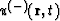move with eikonal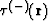it is easy to reconstruct the reflector's location (without picking seismic events) with the help of an operator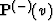. In the case of common source patterns:(67)
and the image of the reflector is a correspondent discontinuity of the field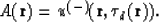(68)

This formula follows directly from equation (60). In order to get a better understanding, let us propose that the discontinuity of the fieldhas an order q. If we differentiate the fieldq+1 times, this discontinuity will become a-function:.If instead of t we substitute, then the support of-function will satisfy equation 60.

In the zero offset case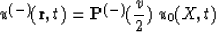(69)
where u0(x,t) is a set of seismic records with s=r=X. The image of the reflector coincides with the discontinuity of the field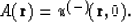(70)

In both cases depth migration consists of two steps:

• reverse wave continuation
• imaging.
This scheme is applicable only in the cases of common source, common receiver, or zero offset gathers. It does not mean that migration cannot be performed for other patterns. On the contrary: we shall show that migration may be done for any arbitrary pattern. But in the previously mentioned gathers, it may be done with these two steps: wave field continuation and imaging. I don't take into consideration possible approximate approaches. Now we can answer the question: why do we have so many algorithms of migration? For this purpose, we can use any kinematically-equivalent operation: all of them are useful for imaging reflectors.

Classical time migration is a result of the substitution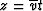:(71)
where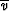is an average velocity along a vertical line and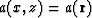.Next: 7: GEOMETRICAL PROBLEMS OF Up: 6: WHY SO MANY Previous: Zero-offset case
Stanford Exploration Project
1/13/1998